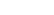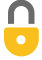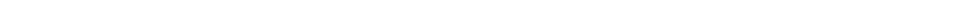Problem 16
Q

# A 0.40-kg iron horseshoe, just forged and very hot (Fig. 14–16), is dropped into 1.25 L of water in a 0.30-kg iron pot initially at $20.0 ^\circ \textrm{C}$. If the final equilibrium temperature is $25.0 ^\circ \textrm{C}$, estimate the initial temperature of the hot horseshoe.Figure 14-16.A
$170^\circ\textrm{C}$In order to watch this solution you need to have a subscription.VIDEO TRANSCRIPT

This is Giancoli Answers with Mr. Dychko. This hot horseshoe made of iron is dropped into a pot of water and the pot is made of iron as well. So, the horseshoe will lose some heat and that'll be the heat gained by the water and the iron pot that contains the water. So, we have, for heat lost we have initial temperature minus final temperature and this is for the horseshoe. So, that's also multiplied by the mass of the horseshoe times its specific heat. And then for the, on the gain side we have the heat gained by the water is the mass of the water times the water specific heat times its final temperature minus its initial temperature plus the mass of the pot times the specific heat of the pot times the final temperature of the pot minus its initial temperature, which is the same as the initial temperature of the water. So, we put a subscript w in there. Or, yeah. So, the pot and the water are initially at the same temperature. So, distributing this into the brackets and expanding the brackets, we have mass of the horseshoe times specific heat of the horseshoe times its initial temperature minus its mass times its specific heat times its final temperature equals this thing just repeated. It didn't do anything to the right hand side there. And then we're gonna solve for initial temperature of the horseshoe. So, we'll take this term to the right hand side, which makes it a plus mass of the horseshoe times specific heat of the horseshoe times the final temperature. And then afterwards divided by mass of the horseshoe and specific heat of the horseshoe. And that gives us the initial temperature of the horseshoe. So, it's the mass of the water which is 1.25 liters times 1 kilogram per liter, density of water there, times specific heat of water, 4,186 joules per kilogram Celsius degree, times the change in temperature of 25 degrees Celsius, final temperature, minus the initial temperature of 20 plus the 0.3 kilograms mass of the iron pot times the specific heat of iron of 450 joules per kilogram Celsius degree times its change in temperature which is the same as that of the water plus 0.4 kilograms, mass of the horseshoe, times the specific heat of iron again since the horseshoe is also made of iron times the horseshoe's final temperature, 25, divided by the mass of the horseshoe, 0.4 kilograms, times the specific heat again of iron. And that gives about 170 degrees Celsius must have been the initial temperature of the horseshoe.

Giancoli Answers, including solutions and videos, is copyright © 2009-2023 Shaun Dychko, Vancouver, BC, Canada. Giancoli Answers is not affiliated with the textbook publisher. Book covers, titles, and author names appear for reference purposes only and are the property of their respective owners. Giancoli Answers is your best source for the 7th and 6th edition Giancoli physics solutions.